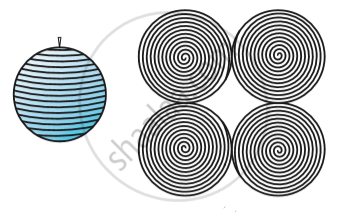# Surface Area of a Sphere

#### Topics

• Surface area of a sphere
• Hemisphere
• Hollow Hemisphere

## Notes

A sheet of paper , draw four circles with radius equal to the radius of the ball . Start filling the circles one by one , with the string you had wound around the ball in following fig.The string, which had completely covered the surface area of the sphere, has been used to completely fill the regions of four circles, all of the same radius as of the sphere.
The surface area of a sphere of radius r = 4 times the area of a circle of radius r = 4 × (π r^2)

So, Surface Area of a Sphere = 4 πr^2

where r is the radius of the sphere.
The curved surface area of a hemisphere is half the surface area of the sphere, which is 1/2 or 4πr^2.

Therefore,
Curved Surface Area of a Hemisphere = 2πr^2
Now taking the two faces of a hemisphere, its surface area 2πr^2 + πr^2

So,
Total Surface Area of a Hemisphere = 3πr^2

If you would like to contribute notes or other learning material, please submit them using the button below.

### Shaalaa.com

Surface Area of a Sphere [00:03:04]
S
0%